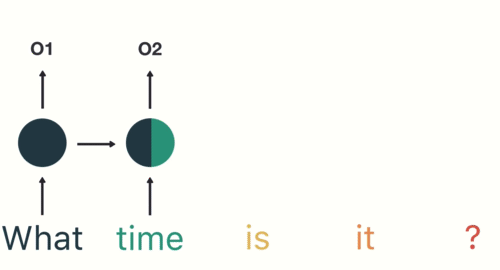# Depth-Gated LSTM: From A to Z!# Depth-Gated LSTM: From A to Z!

Recurrent Neural Networks(RNN) suffer from short-term memory. This means that if there is a long sequence, an RNN will have a problem in carrying information from earlier time steps to later ones. Which may force the RNN to leave out important information from the beginning.

In-depth, during backpropagation, recurrent neural networks suffer from the vanishing of its gradients(Gradients are values used to update neural network weights)this problem occurs when the gradient shrinks as it back propagates through time.

# But First, What is an RNN?

Recurrent Neural Network(RNN) is a type of Neural Network where the output from the previous step is fed as input to the current step. In other words, it converts the independent activations into dependent ones by providing the same weights and biases to all the layers, thus reducing the complexity of increasing parameters and memorizing each previous outputs by giving each output as input to the next hidden layer.

# RNN & LSTM

Long Short Term Memory network(LSTM) is a special kind of RNN, it is capable of learning long-term dependencies. They were introduced by Hochreiter & Schmidhuber (1997) and were refined and popularized by many people.

LSTMs are designed to avoid the long-term dependency problem. This means that they can remember information for long periods of time which gives them the ability not to struggle to learn!

All recurrent neural networks have the form of a chain of repeating modules of the neural networks. In standard RNNs, this repeating module will have a very simple structure, such as a single tanh layer.

Their cell state is kind of like a conveyor belt. It runs straight down the entire chain, with only some minor linear interactions. So it’s very easy for information to just flow along with it unchanged.

The LSTM has the ability to remove or add information to the cell state, carefully regulated by structures called gates.

Gates are a way to optionally let information through. They are composed out of a sigmoid neural net layer and a pointwise multiplication operation.

# So, Can we consider the LSTM as an efficient solution?

The LSTM module, which today can be seen as multiple switch gates, can bypass units and thus remember for longer time steps. LSTM has a way to remove some of the vanishing gradient’s problems.

But not all of it, Still, we have a sequential path from older past cells to the current one. In fact, the path is now even more complicated because it has additive and forgets branches attached to it.

# How is It possible For an LSTM To Perform Better?

Applying an extension of long short-term memory (LSTM) neural networks to using a depth gate to connect memory cells of adjacent layers. Doing so introduces a linear dependence between lower and upper layer recurrent units. This linear dependence is gated through a gating function, which we call depth gate. This gate is a function of the lower layer memory cell, the input to, and the past memory cell of this layer.

# This is called the depth-gated LSTM(DGLSTM).

Where The depth-gate controls how much flow from the lower memory cell directly to the upper layer memory cell.Mathematically,

b(L+1)d is a bias term. W(L+1)xd is the weight matrix to relate the depth gate to the input of this layer. The past memory cell is also related via a weight vector w(L+1)cd. To relate the lower layer memory, it uses a weight vector w(L+1)ld. Note that, if lower and upper layer memory cells have a different dimension, w(L+1)ld should be a matrix instead of a vector.

In DGLSTM, equations are the same as the standard LSTM, except that
DGLSTM uses a superscript L + 1 to denote operations at layer L + 1.
The idea of using gated linear dependence can also be used to connect the first layer memory cell c(1)t with the feature observation x^(0).

# Results

The first is BTEC Chinese to English machine translation
task. Its training set consists of 44016 sentence pairs. We use its devset1 and devset 2 for validation, which in total have 1006 sentence pairs. We use its devset3 for tests, which has 506 sentence pairs.

The second dataset is PennTreeBank (PTB) for language modeling. It consists of 42075 sentences for training, 3371 sentences for development, and 3762 sentences for a test.

# As a conclusion,

The depth-gated LSTM architecture uses a depth-gate to have a gated linear
connection between lower and upper layer memory cells. We observed better performances using this new architecture. So, Do you think that this is going to be the solution to the LSTM’s problems?is it going to be a state of art?cannot wait t hear your thoughts!

Experiments results source: The official paper of the Depth gated LSTM